Next: Modeling Cortical Oscillations Up: Synchronous Oscillations Based on Previous: Introduction

# Model Description

As the building block, the model of a single oscillator is defined in the simplest form as a feedback loop between an excitatory unit and an inhibitory unit (Figure 1a):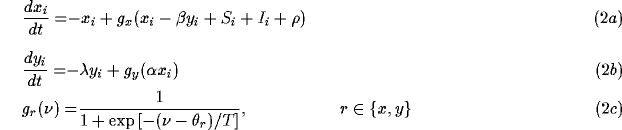where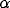and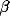are positive parameters describing the coupling between the two units. Adding an inhibitory feedback to the inhibitory unit as in [63,71] does not seem to change the qualitative behavior.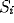represents the input from other oscillators and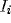represents external stimulation.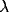is a decay parameter, and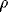denotes the amplitude of a Gaussian noise term.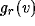is a sigmoid gain function with threshold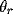, where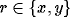, and parameter T.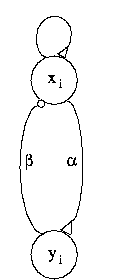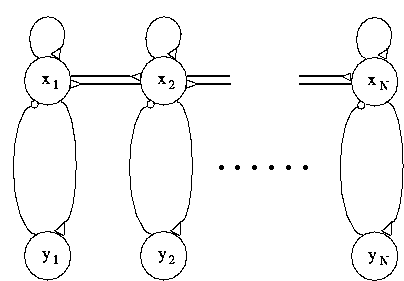(a) (b)

Figure 1: ( a) Basic oscillator model formed by a feedback loop between an excitatory unit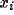and an inhibitory unit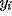.andare mutual connection strengths. ( b) A chain of N oscillators. Small triangles indicate excitatory connections, and small circles inhibitory connections.

Equation 2 is essentially a simplification of the Wilson and Cowan oscillators , and it has been shown that the system produces oscillations within a wide range of parameters [3,72]. Figure 2 shows a typical limit cycle drawn on the x-y plane, as exhibited by a single oscillator. The two nullclines split the x-y plane into four regions. Within each region,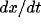and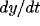have unique signs. For example,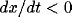and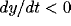in the upper-left region (where the starting point lies in). Thus, both x and y decrease in this region until the trajectory intersects with the x-nullcline. After the intersection, the trajectory moves into the lower-left region where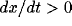and thus x starts to increase. Following this type of qualitative analysis, one can see why (2) gives rise to oscillations. As in the following simulations, the equations were numerically solved with the simple Euler method, where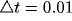. The results were also confirmed using the fourth-order Runge-Kutta method. The oscillator model can be biologically interpreted as a mean field approximation to a network of excitatory and inhibitory neurons [7,23,56].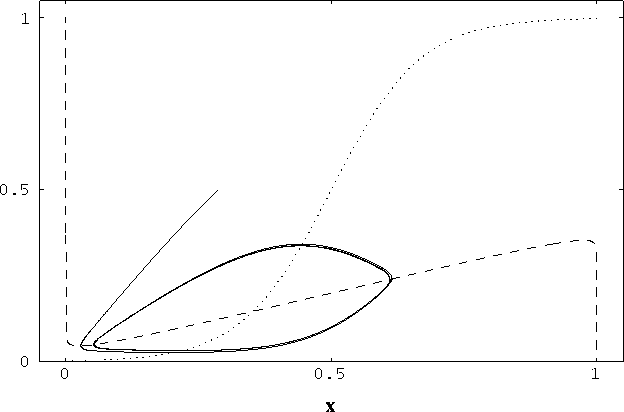Figure 2: Nullclines and limit cycle trajectory of a single oscillator as shown in the phase plane. The x-nullcline (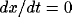) is shown by the dashed curve and the y-nullcline (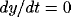) is shown by the dotted curve. The oscillator started from a randomly generated point, the end point near (0.3, 0.5), and it quickly fell in the trajectory of a limit cycle. The parameters for this simulation are= 0.3,= 2.5,= 0.01,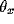= 0.2,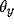= 0.15, T = 0.025,= 1.0, and I = 0.2. 2,000 integration steps.

To see the detailed behavior of an isolated oscillator, Figure 3 presents the simulation of the system with different parameters. Figure 3a shows that if the external input is very small, the oscillator will be silent; but if the input is very high the system reaches a saturation point. Oscillations (limit cycles) occur between the two extremes. In other words, oscillations are driven by input, as opposed to the phase model where oscillation is built into the system. Figure 3b shows thatcontrols the frequency of oscillations, and Figure 3c demonstrates thathas a major influence on the amplitude of oscillations.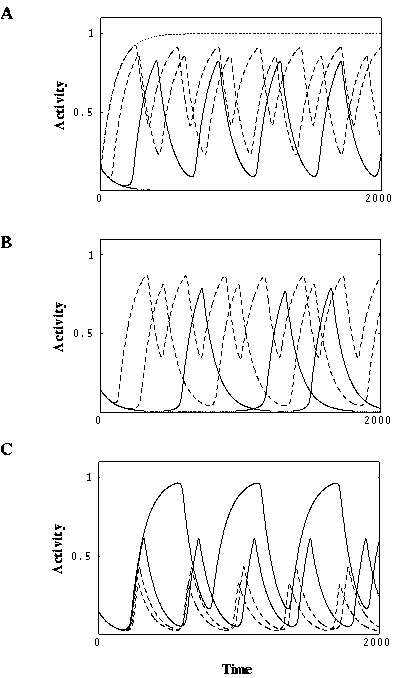Figure 3: Behavior of a single oscillator. ( a) Effect of varying external input. Solid thick, I = 0.0; solid thin, I = 0.2; dashed thick, I = 0.4; dashed thin, I = 0.8; dotted, I = 1.6.= 1.0 and= 0.2. ( b) Effect of varying. Solid thick,= 0.2; solid thin,= 0.4; dashed thick,= 0.8; dashed thin,= 1.6.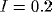and= 0.2. ( c) Effect of varying. Solid thick,= 0.15; solid thin,= 0.3; dashed thick,= 0.45; dashed thin,= 0.6.and= 1.0. The other parameters are the same as in Figure 2. 2,000 integration steps.

Weak coupling between oscillators (is relatively small) does not disrupt the oscillatory behaviors of individual oscillators. To study the properties of a network of oscillators, first a chain of N oscillators is constructed with only neighboring coupling between excitatory units, as shown in Figure 1b. We define the coupling as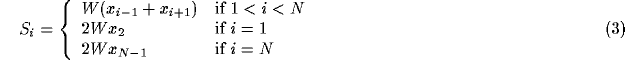where W is a connection weight. Note that the weights of the connections to the two end oscillators 1 and N double those of the connections to the other interior ones in the chain. We found, with uniform external input and random values forand(namely random phases) initially, that the chain with coupling (3) is synchronized after an initial period of rapid phase transitions. The synchronization is absent, however, if the connections to the end oscillators are equally strong as to the interior ones. Instead we found phase shifts across the chain. Figure 4presents a simulation with N = 30. Note that there were small phase differences when nearly stable limit cycles started to occur, but the differences diminished as time went on.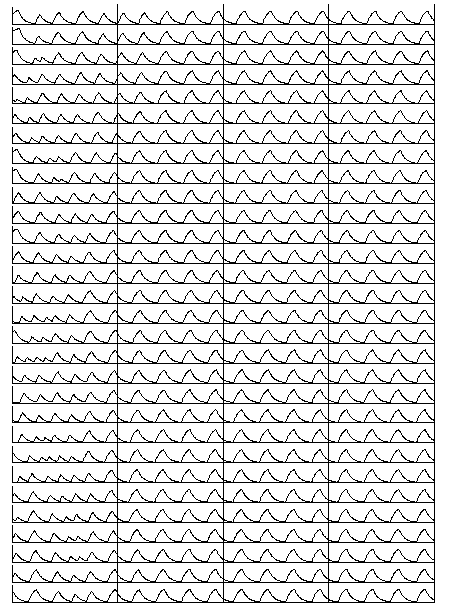Figure 4: Synchrony in a chain of oscillators. The input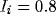, and the initial values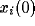and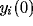were randomly generated within the range [ 0.8,0]. The height of the ordinate of each oscillator is 1.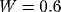and N = 30. The parameters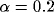,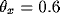, and the remaining ones are the same as in Figure 2. 8,000 integration steps. Vertical lines are drawn to help identify phase relations among the oscillators.

A chain of oscillators using the phase model has been extensively studied for modeling swimming behaviors in fish [11,33]. Cohen et al.  noted that phase-locking with no phase shift can be reached with a chain of identical oscillators. However, phase-locking cannot be produced if there is inhomogeneous input to a chain, contradicting the experimental conditions of Gray et al , where synchrony can occur even if two stimulus bars are not connected (more discussion in the Modeling Cortical ... section). For this reason, the chain model was considered not proper for modeling the phase locking experiments . But, as will be clear later, our model does not suffer from this problem.

(3) is not a necessary condition for phase-locking. Let us call an oscillator active if it receives an external stimulus. We observed that in a system defined by (3), as long as the overall (sum of) weights of the connections converging on every active oscillator from all other active oscillators are kept constant, phase-locking occurs. This condition is called the equal weight condition. (3) is a special case of this condition. It is easy to show that without noise (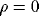) homogeneous input leads to the solution of synchronized oscillations to (2). When the system is in synchrony,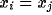, for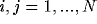. With the equal weight condition, we have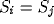, and thus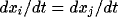, and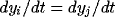, for. Therefore, the system will keep the synchrony in its evolution. The stability of the synchronized solution remains to be analyzed. Extensive numerical simulations have been conducted, however, and we found that the system is stable with respect to perturbations by noise once it reaches synchronous oscillations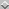. Intuitively, positive coupling between neighboring oscillators serves to drive the oscillators close to each other in phase and it can also correct small discrepancies among the phases of the oscillators. We have tested one dimensional chains of up to 256 oscillators and two dimensional grids of up to 100 by 100, and synchrony in such systems is stable. So even the conclusion concerning long range synchrony might not be established when the size of the network tends to infinity, the significance of the system studied here does not vanish because almost all practical applications of oscillator networks, such as image analysis (see the Why Local ... section), require only limited sizes.

The equal weight condition is easily achieved if one allows connection weights to be dynamically modified on a fast time scale, an idea first introduced by von der Malsburg . In this scheme, there is a pair of connection weights from oscillator j to i, one permanent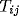, and another dynamic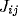(so called Malsburg synapses, see ). Permanent links reflect the hardwired structure of a network, while dynamic links quickly change from time to time. In computations, though, only dynamic links formed on the basis of permanent links play an effective role. The equal weight condition can be naturally realized by a modification rule of dynamic links which combines a Hebbian rule  that emphasizes coactivation of oscillators i and j and a normalization of all incoming connections to an oscillator. More specifically, it can be implemented by a two-step procedure: First update dynamic links and then normalization: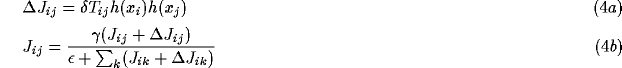whereand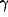are positive parameters, and function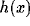measures whether x is active. It is here simply defined as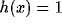if <x> is greater than a constant and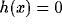otherwise, where the angular bracket <x> stands for temporal averaging of the activity x.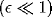is introduced to prevent division by zero. Note that weight normalization of this form is commonly used in neural network models for competitive learning [22,61].

With introduction of fast changing synapses, the equal weight condition in (3) can now be reached by dynamics in (4) from a natural condition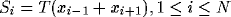, and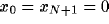defined for permanent links, where T is the strength of every permanent link. In other words, the same permanent link is established for only neighboring oscillators, and there is no permanent connection beyond nearest neighbors. Because of this, dynamic links can be established for only neighboring oscillators according to (4a). Since the entire chain is stimulated,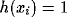for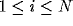. Following (4), after a very brief beginning period,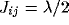if oscillators i and j are nearest neighbors and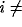1 or N. Additionally,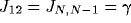. This connection pattern of dynamic (effective) links is equivalent to (3) if one lets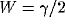.

It should be obvious that the result concerning global synchrony extends to oscillator networks of higher dimensions than 1-D. A prototype of 2-D network is illustrated in the Modeling Cortical ... section. Also, the result is established for lateral connections beyond nearest neighbors. Indeed, more extensive connections can speed up the synchronization process. In a sense, synchrony in fully connected networks is a special case of our result based on local coupling.Next: Modeling Cortical Oscillations Up: Synchronous Oscillations Based on Previous: Introduction